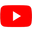#### Not many great matches came back for your search: "ZC-6726"

• Try more general keywords
• Try fewer keywords
23+5=28×2=56 · 6+7=13×2=26 · 5+2=7×2=14 · 7+5=12×2=24 · So 24 is the ans Answer from Akash Rana on examcompetition.comZC